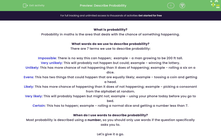# Describe Probability

In this worksheet, students will practise using words to describe probability.Key stage:  KS 4

Year:  GCSE

GCSE Subjects:   Maths

GCSE Boards:   AQA, Eduqas, Pearson Edexcel, OCR,

Curriculum topic:   Probability

Curriculum subtopic:   Probability Basic Probability and Experiments

Difficulty level:#### Worksheet Overview

What is probability?

Probability in maths is the area that deals with the chance of something happening.

What words do we use to describe probability?

There are 7 terms we use to describe probability:

Impossible: There is no way this can happen;  example - a man growing to be 200 ft tall.

Very unlikely: This will probably not happen but could; example - winning the lottery.

Unlikely: This has more chance of not happening than it does of happening; example - rolling a six on a dice.

Evens: This has two things that could happen that are equally likely; example - tossing a coin and getting a head.

Likely: This has more chance of happening than it does of not happening; example - picking a consonant from the alphabet at random.

Very likely: This will probably happen but might not; example - using your phone today before you go to bed.

Certain: This has to happen; example - rolling a normal dice and getting a number less than 7.

When do I use words to describe probability?

Most probability is described using a number, so you should only use words if the question specifically asks you to.

Let's give it a go.

### What is EdPlace?

We're your National Curriculum aligned online education content provider helping each child succeed in English, maths and science from year 1 to GCSE. With an EdPlace account you’ll be able to track and measure progress, helping each child achieve their best. We build confidence and attainment by personalising each child’s learning at a level that suits them.

Get started